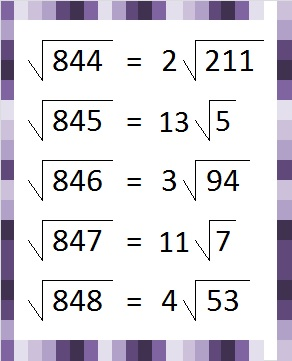# Let’s revisit √844

844 is repdigit 444 in BASE 14 because 4(14²) + 4(14¹) + 4(14⁰) = 844.

None of the following should surprise us:

• Since 844 is 444 in BASE 14, we know 4 is a factor of 844.
• (844)/2 = 422 which is 222 in BASE 14.
• (844)/4 = 211 which is 111 in BASE 14

Back when I wrote Post 266 I made some observations about the numbers 844, 845, 846, 847, and 848. Those are the smallest five consecutive numbers whose square roots can be simplified. Now that I’m writing Post 844, I am just as amazed by those five consecutive numbers and their square roots.Although I included that graphic and the next one in that previous post. Both of them are worth a second look.• 844 is a composite number.
• Prime factorization: 844 = 2 × 2 × 211, which can be written 844 = 2² × 211
• The exponents in the prime factorization are 2 and 1. Adding one to each and multiplying we get (2 + 1)(1 + 1) = 3 × 2  = 6. Therefore 844 has exactly 6 factors.
• Factors of 844: 1, 2, 4, 211, 422, 844
• Factor pairs: 844 = 1 × 844, 2 × 422, or 4 × 211
• Taking the factor pair with the largest square number factor, we get √844 = (√4)(√211) = 2√211 ≈ 29.051678